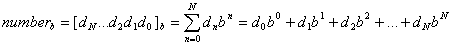Showing posts with label Numeral system. Show all posts
Showing posts with label Numeral system. Show all posts

## Numeral system

A numeral system (or system of numeration) is a writing system for expressing numbers, that is, a mathematical notation for representing numbers of a given set, using digits or other symbols in a consistent manner. It can be seen as the context that allows the symbols "11" to be interpreted as the binary symbol for three, the decimal symbol for eleven, or a symbol for other numbers in different bases.
Read Bengali version click here....
The number the numeral represents is called its value.

Ideally, a numeral system will:

Represent a useful set of numbers (e.g. all integers, or rational numbers)
Give every number represented a unique representation (or at least a standard representation)
Reflect the algebraic and arithmetic structure of the numbers.

For example, the usual decimal representation of whole numbers gives every non zero whole number a unique representation as a finite sequence of digits, beginning by a non-zero digit. However, when decimal representation is used for the rational or real numbers, such numbers in general have an infinite number of representations, for example 2.31 can also be written as 2.310, 2.3100000, 2.309999999..., etc., all of which have the same meaning except for some scientific and other contexts where greater precision is implied by a larger number of figures shown.

Numeral systems are sometimes called number systems, but that name is ambiguous, as it could refer to different systems of numbers, such as the system of real numbers, the system of complex numbers, the system of p-adic numbers, etc. Such systems are not the topic of this article. Reed More .....
source-http://en.wikipedia.org/wiki/Numeral_system

### Type of Number System

• Binary Numeral System
• Octal Numeral System
• Decimal Numeral System
• Hex Decimal Numeral System

### Numeral Systemb - numeral system base
dn - the n-th digit
n - can start from negative number if the number has a fraction part.
N+1 - the number of digits

### Binary Numeral System - Base-2

Binary numbers uses only 0 and 1 digits.
B denotes binary prefix.

#### Examples:

101012 = 10101B = 1×24+0×23+1×22+0×21+1×20 = 16+4+1= 21
101112 = 10111B = 1×24+0×23+1×22+1×21+1×20 = 16+4+2+1= 23
1000112 = 100011B = 1×25+0×24+0×23+0×22+1×21+1×20 =32+2+1= 35

### Octal Numeral System - Base-8

Octal numbers uses digits from 0..7.

#### Examples:

278 = 2×81+7×80 = 16+7 = 23
308 = 3×81+0×80 = 24
43078 = 4×83+3×82+0×81+7×80= 2247

### Decimal Numeral System - Base-10

Decimal numbers uses digits from 0..9.
These are the regular numbers that we use.

#### Example:

253810 = 2×103+5×102+3×101+8×100

### Hexadecimal Numeral System - Base-16

Hex numbers uses digits from 0..9 and A..F.
H denotes hex prefix.

#### Examples:

2816 = 28H = 2×161+8×160 = 40
2F16 = 2FH = 2×161+15×160 = 47
BC1216 = BC12H = 11×163+12×162+1×161+2×160= 48146

Loading...

## Feature Article

• - The Bengali Calendar or Bangla Calendar (বঙ্গাব্দ Bônggabdô or Banggabda) is a solar calendar used in the region of Bengal. A revised version of the calend...